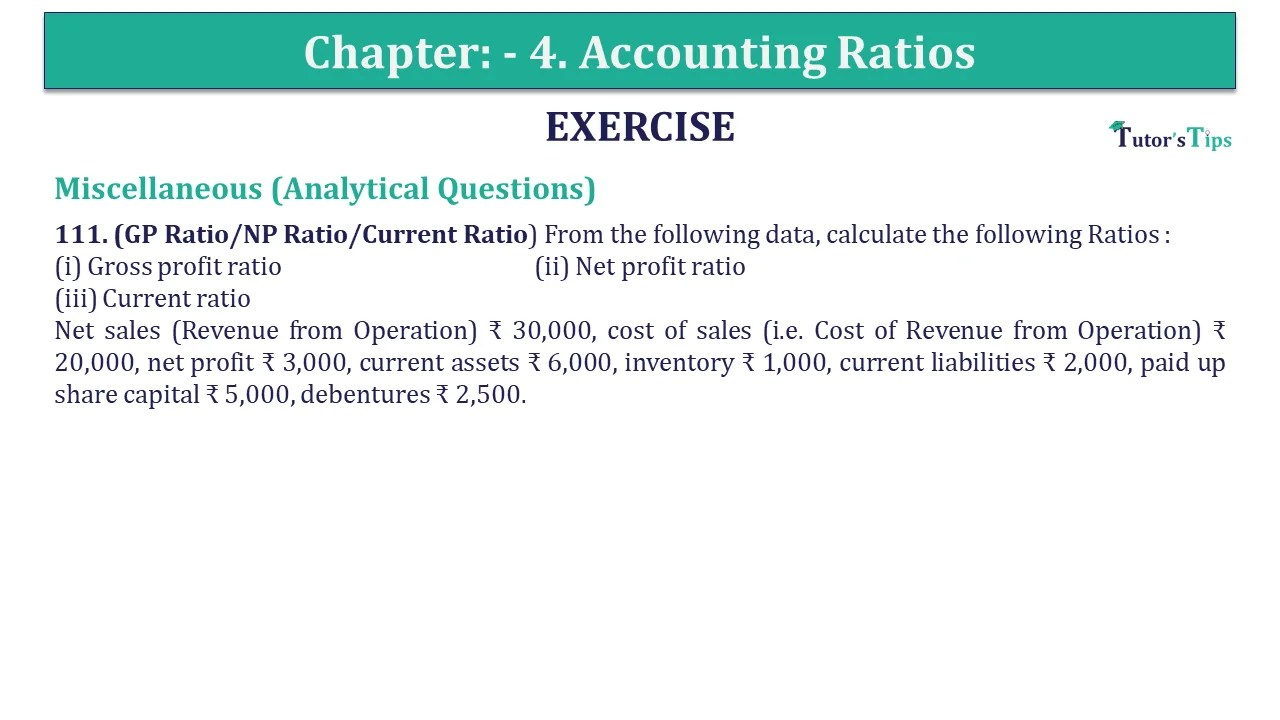# Question 111 Chapter 4 of +2-B – USHA Publication 12 ClassQ-111- CH-4 Book 2 - Usha Pub. +2 Book 2020 - Solution

Question 111 Chapter 4 of +2-B

Miscellaneous (Analytical Questions)

111. (GP Ratio/NP Ratio/Current Ratio) From the following data, calculate the following Ratios :
(i) Gross profit ratio
(ii) Net profit ratio
(iii) Current ratio
Net sales (Revenue from Operation) ₹ 30,000, cost of sales (i.e. Cost of Revenue from Operation) ₹ 20,000, net profit ₹ 3,000, current assets ₹ 6,000, inventory ₹ 1,000, current liabilities ₹ 2,000, paid up share capital ₹ 5,000, debentures ₹ 2,500.

## The solution of Question 111 Chapter 4 of +2-B: –

 (i) Gross Profit Ratio = Gross Profit X 100 Net Sales = ₹ 10,000 X 100 ₹ 30,000 = 33.33%

 (ii) Net Profit Ratio = Net Profit X 100 Net Sales = ₹ 3,000 X 100 ₹ 30,000 = 10%
 (iii) Current Ratio = Current Assets Current Liabilities = ₹ 6,000 ₹ 2,000 = 3: 1

Working Notes :

 Gross Profit = Net Sales – Cost of goods sold = ₹ 30,000 – ₹ 20,000 = ₹ 10,000

Also, Check out the solved question of previous Chapters: –